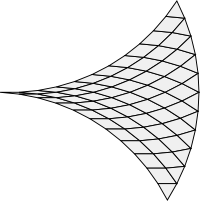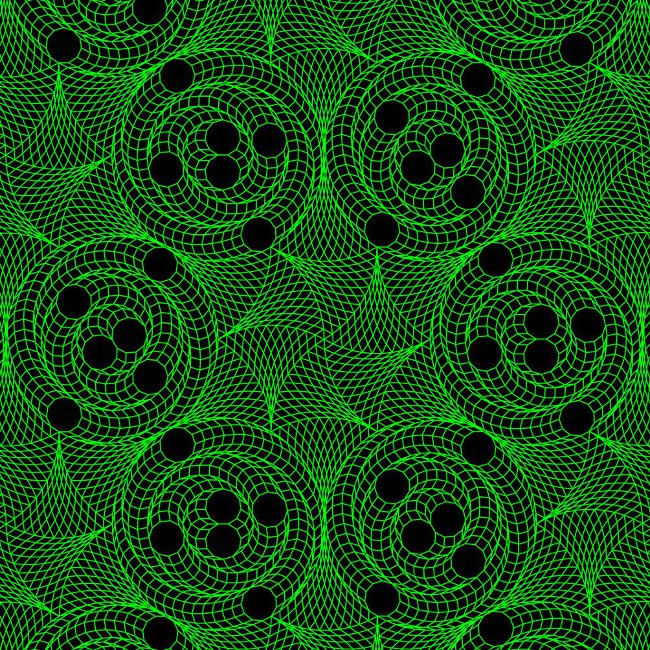# Part 3: A universal tiler

'The adventures first,' said the Gryphon in an impatient tone: 'explanations take such a dreadful time.'

As mentioned in the section The forbidden tilings, tilings of the plane using regular polygons alone are restricted to triangles, squares, hexagons, dodecagons and the one unique octagon 4.8.8 uniform tiling. Adding rhombs to the prototile set allows a much richer variety of tilings including all the "forbidden" vertex figures. This leads to tilings including pentagons, heptagons, nonagons, decagons and even larger polygons such as the 42-gon.The rhombic triangle can be used to construct a universal tiler.

But are all the possible tilings involving rhombs and regular polygons restricted to the regular polygons that appear in the 21 possible regular polygon vertex figures?

No.

In fact, amazingly, there is an algorithm to efficiently construct a translational unit for a periodic tiling of the plane from rhombs, triangles and any regular polygon!

The existence of an efficient universal tiling algorithm for regular polygons is surprising because many such general problems in tiling theory turn out to be undecidable or to require time-consuming NP-complete algorithms. For example, in 1966 David Berger showed that no algorithm could exist to automatically construct tilings from even simple prototile sets of Wang tiles.

However, the situation for rhombs and regular polygons is different. Sampath Kannan and Danny Soroker (1992) and Richard Kenyon (1993) independently developed an efficient algorithm to decompose certain finite simple polygons into rhombs (and more generally, parallelograms), and a modification of this algorithm can be used to construct periodic plane tilings also involving regular polygons as explained in the following sections.

Here's an example involving hendecagons (11-sided regular polygons) constructed using a prototile set that also includes rhombs and triangles:I've created a larger 1280x1024 image of this beautiful tiling.

In the next few sections I'll describe the algorithm and provide the mathematical background for a universal regular polygon tiler.